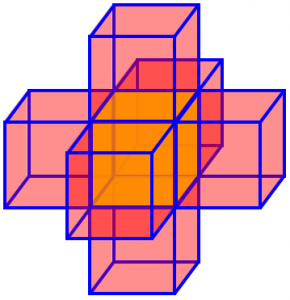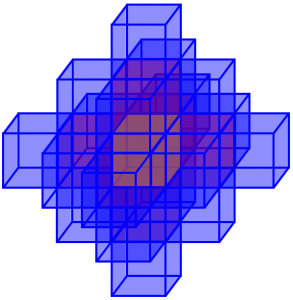# Building Patterns and Sequences

In the past (last May and November), we discussed ways to find patterns or sequences in numbers, sometimes leading to a formula. This included an example where the sequence turned out not to be just a provided list of numbers, but a process that generated the numbers. I want to focus on that type of problem this time; most of the examples we have happen to involve arrangements of cubes.

## Hidden faces: all in a row

How Many Hidden Faces?

I have to do an investigation at school on hidden faces. When you look at a cube you cannot see one face - this face is hidden. So if you have one cube then two cubes all in a straight line up to eight you will work out a pattern

1-1 2-4 3-7 4-10 5-13 6-16 ...

Can you please find a formula to find out the number of hidden faces, eg. 3n-2?  I also need proof.  Can you please help me? I've spent 5 hours on it already!

What Amy means by “hidden” faces is faces of cubes that are not visible from any direction, because they are “glued” to another cube, or because they are on the table: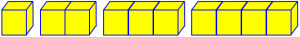Amy had correct data, and a correct formula, as it turns out:

• 1 cube: 1 hidden face (bottom), 5 visible
• 2 cubes: 4 hidden faces (2 on bottom, 2 between cubes), 8 visible
• 3 cubes: 7 hidden faces (3 on bottom, 4 between cubes), 11 visible
• 4 cubes: 10 hidden faces (4 on bottom, 6 between cubes), 14 visible
• 5 cubes: 13 hidden faces (5 on bottom, 8 between cubes), 17 visible
• 6 cubes: 16 hidden faces (6 on bottom, 10 between cubes), 20 visible

What she needed was to be able to convince someone (perhaps herself) that she was right: a proof. I replied, first clarifying the statement of the problem:

Actually, if I look at a cube I can see only three of its faces at one time; I presume you mean that it is sitting on a table and you are allowed to look all around; only the face in contact with the table (or with another cube) is "hidden".

One way to approach this kind of problem is to think about how things change from one step to the next. If I have a row of cubes, say your three in a row with 7 hidden, and add another to the end, how many new hidden faces are there? There's the one on the end of the row you had, which is now covered and becomes hidden; and there are two on the new cube that will be hidden, one on the bottom and one that touches the row. So each time you add a cube, you add three more hidden faces. Sounds like an arithmetic sequence to me.

Another approach is to think about one whole row and break the hidden faces down into groups. There are the faces on the bottom (one per cube) and the faces between cubes (two wherever a pair of cubes meet). That easily gives you a formula.

The second approach is what I used in doing my counting above. We can see that with n cubes, there are n bottom faces, and $$2(n-1)$$ interior hidden faces, giving us the formula $$H = n + 2(n-1) = 3n – 2$$. This is reminiscent of what we talked about in the post Counting Faces, Edges, and Vertices, finding an organized way to count.

The first approach tells us that we start with 1 for n = 1, and add 3 for each of $$n-1$$ cubes we add, giving the formula $$H = 1 + 3(n-1) = 3n – 2$$. It’s always encouraging when we get the same result two different ways.!

What if we had been asked for the number of visible faces? We could use either of these ways to find it directly; or, having found the number of hidden faces, we could subtract that from the total number of faces on n cubes: $$V = 6n – (3n – 2) = 3n + 2$$. (Interesting – there are always 4 more visible faces than hidden faces!) You can check this against the numbers I listed above.

For another example of the same sort of problem, see

Visible/Hidden Sides on Stacked Cubes

## Hidden faces: three by three

Here is a more complicated question of the same type, from 2001:

Hidden Faces of Cubes

My daughter has to produce an equation to show the number of hidden faces when three rows of cubes are placed together on a flat surface.  The data look like this

Number of Cubes(n)     Hidden Faces(h)
3                      7
6                     20
9                     33
12                     46
15                     59
18                     72
21                     85
24                     98
27                    111
30                    124

I've worked out the difference between n squared and h, and the differences between these results. The difference between the differences I calculate as 18 constantly, so the next set of differences will obviously be 0. I can't see how to get a formula from this, though, and am not sure if I'm heading in the right direction. I haven't done algebra for 20 years and used to hate it, but I must say I'm quite enjoying this problem!

Yes, math is more fun when you do it because you find it interesting!

I think a better description would be, “when rows of three cubes are placed together”, though I can also see it Nigel’s way. It looks something like this: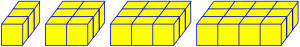Here again, a “hidden” face is one that is either on the table or against another cube. For n = 1, there are 3 faces on the table (the bottom) and two pairs glued together, for a total of 7 hidden (and 11 visible).

Nigel has done some of the things discussed in the previous posts, Pattern and Sequence Puzzles and Pattern and Sequence Puzzles Revisited. He compared each term in his list (which must have taken a lot of work to compile!) with the square, and he also used another technique (the method of finite differences, which will be the subject of some posts here eventually) to determine that the sequence has a quadratic formula. Unfortunately, he’s wrong about that, apparently because he started with the differences between two related sequences, rather than between successive terms of one sequence.

I began by commenting on what he had done, and what he might do to correct it:

Before I get started, I should ask whether you are doing this, or your daughter. I hope you are giving her opportunities to learn from this as you work together; learning together is great!

You haven't told me how old she is; I'll assume she is learning algebra, so what you are doing is relevant to her.

The approach you are taking, analyzing the data after the fact to find a formula that fits it, uses a method called finite differences, which is explained in our archives; here is one such page:

Method of Finite Differences
http://mathforum.org/dr.math/problems/gillett.10.12.00.html

I recommended not starting with the numbers:

But I don't like this method for a problem like yours. Why? Because when you get a formula, all you will know is that it fits the particular data you used. It doesn't tell you whether you made a mistake in your numbers, or whether the pattern will continue when the numbers get larger. And in math, I like to KNOW, not just ASSUME.

If she was told to use this method (and was told how), then it is fine to use it; and you will certainly enjoy learning the method anyway. But here's how I would prefer to do the problem: rather than looking at the data you gathered, I would look at HOW you gathered it, and find the pattern BEHIND the numbers.

So what causes hidden faces, and how do they grow?

Since we always have a multiple of 3 cubes, I started by simplifying the variable, and then using the first method of the answer above:

I'm going to start out talking not about the number of cubes, but the number of columns of three, so that all natural numbers will work, not just multiples of three. With one column

c = 1

X
X
X

there are three hidden faces on the bottom (one per cube), and four between cubes (two per pair of adjacent cubes). This gives seven in all.

When I add a second column

c = 2

X X
X X
X X

I have added another seven under and between the new cubes; I have also added six more, between the old and new columns (two per pair of adjacent cubes). So my new total is 7+7+6 = 20.

This could be something like what Nigel did to get his table.

Now each time I add another column, the same thing will happen, and I will add 7+6 more hidden faces. (In other similar problems, it will be a little more complicated.)

So for c = 1, I have 7, and for each increase of 1, I add 13. This is a linear equation:

H = 7 + 13(c-1)

since with c columns I have added c-1 columns to the first.

Since my c is 1/3 of your n, your formula (for n a multiple of 3) will be
H = 13c - 6 = 13n/3 - 6

Go ahead and try the other method, and verify that it works. Then try some more complicated patterns, such as making layers of cubes in a three-dimensional block.

The method I used here to write the equation is the point-slope form for an equation of a line, $$y – y_1 = m(x – x_1)$$. When x is 1, y is 7, so the line passes through $$(x_1, y_1) = (1, 7)$$; and the slope (increase in y per unit increase in x) is 13. You can also think of this as starting with 7, and then adding 13 for each of c – 1 steps to get from 1 to c.

We can also check this formula against Nigel’s data. When n = 30, the last row of his table, we get $$H = \frac{13(30)}{3} – 6 = 124$$, just what he found.

For a similar count (of cubes put together to make a large cube), see

Hidden Faces in a Set of Cubes

Let’s look at one more from 2002, this time not looking for hidden faces, but for the number of cubes added at each step. This one needs a little background: Kathy had initially just given us a sequence and her attempt at a formula, asking why the formula didn’t work for the first term; what follows is her restatement of the question after we asked for the context of the question:

Finding the nth Term

This is the investigation. This was the starting point:

A colony of bees is making a honeycomb consisting of hexagonal cells. They start with the center cell. The next day they add the six cells round the center cell, and on the third day they add the remaining ring of cells in the picture. Every day they add a new ring of cells right round the honeycomb.

The investigation posed two questions:
1. How many cells will there be at the end of the sixth day?
2. On which day will the number of cells pass the 1000 mark?

I managed to find the formula for this.
I extended this by using 3D shapes instead of 2D shapes. I used cubes, with day 1 starting with 1 cube; on day 2 I surrounded the first cube with more cubes, both the sides and edges, completely surrounding it.  I.e., making a 3x3x3 cube on day 2.

I continued this for each day: 3x3x3, 5x5x5, 7x7x7, etc..  I wanted to find the relation between the number of days and the number of NEW ADDITIONAL cubes. These are the results I got:

Number of days(n)	New additional days(y)
1                         0
2                        26
3                        98
4                       218
5                       386

The formula I  got was: Y = (2n-1)^3-(2n-3)^3

This works for all except the first day! I would be grateful for any help you give me.

Kathy is my kind of student — taking an interesting question and extending it in two directions (no pun intended): making it 3-dimensional, and asking a different question. Though she didn’t ask about it, let’s take a quick look at the honeycomb question first. We have something like this, where each color represents a new day’s cells: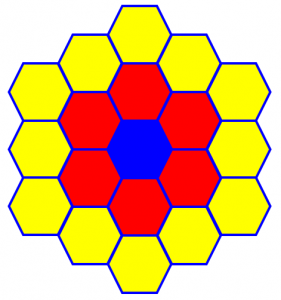Our sequence representing the total number of cells starts off 1, 7, 19, … , which turns out to follow the formula $$a_n = 3n^2 – 3n + 1$$. (See Centered Hexagonal Number.) If the problem had been to count the number in each layer, we would have the sequence 1, 6, 12, …, adding 6 each time except for the first, which would be the arithmetic sequence 0, 6, 12, … if it weren’t for the first term being 1 instead. So this problem would have raised the same issue Kathy raised in her own problem. It was the choice to count the new cubes (or hexagons) that caused her issue.

I responded to the cube problem: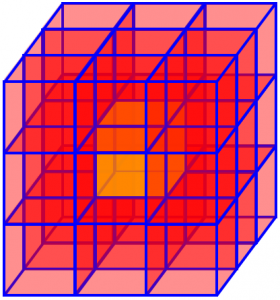I would have said that on day 1 you have added 1 block, rather than zero, since you probably had none before beginning.

Further, since on each day the total is a complete cube, as on the first and second days, the total on day N is (2N-1)^3, so the number added on day N would be

(2N-1)^3 - (2(N-1)-1)^3

which is just what you got. (I see now that you did not get this formula from the numbers, but directly from the problem, which is good!) This can be simplified to

[8N^3 - 12N^2 + 6N - 1] - [8N^3 - 36N^2 + 54N - 27] =

24N^2 - 48N + 26

Does this formula agree with the data (after correcting the first line from 0 to 1)?

I would check this by making a table including the total each day:

day     total     new   formula
0     0^3=  0      -       -
1     1^3=  1      1       2
2     3^3= 27     26      26
3     5^3=125     98      98
4     7^3=343    218     218

Hmmm … no, the formula is still off.

Now, we can see why day 1 would be anomalous: on the other days, we started with a cube, and added two to each dimension, going to the next odd cube. But on the first day, we didn't start with a cube -1 unit on a side; we started with nothing, and added a single cube. That is, the number on the first day did not arise by following the same rule of surrounding an existing cube, so it is not surprising that your formula doesn't apply.

Notice that my formula for the _total_ applies to day 1, but not to my fictional day 0; so that your formula for the number of blocks added applies only _after_ day 1, since it depends on the previous day's total.

Is this a problem?

It is not unusual in mathematics to require a starting condition apart from the formula that applies elsewhere. You just have to say that the formula holds for N > 1. In some cases it makes some sort of sense to continue the pattern back in time before you started, but here that doesn't work, so we don't need to be troubled by the formula not being extensible to N < 2.

Real life formulas commonly have a “piecewise” aspect to them; and that, I think, is the main answer to Kathy’s question.

Incidentally, I don't think your description of the process by which you are building larger blocks is quite right. You are not just adding to the faces and edges, but also to the corners. If you added only cubes that touch on a face, you would have a considerably more interesting problem, since the shapes you are building would not be mere cubes.
But the question you raised turns out to be very interesting, and deserves the thought we've given it! It's all too easy to ignore starting conditions and imagine that all formulas work for all cases, and checking against reality is a very good practice to avoid this.